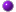﻿ The Number 11 in Mathematics and Computers -

## Interesting Things About The Number 11 in Computers and Mathematics

• 11 is the first number which cannot be represented by a human counting with his or her eight fingers and two thumbs additively.

• 11 is the fourth number that stays the same when written upside down. The first three are 0, 1 and 8.

• If a number is divisible by 11, reversing its digits will result in another multiple of 11

• 111111111 x 111111111 = 12345678987654321 (Forms a Pyramid)

• Pi can be expressed by an improper fraction; 22/7 (22 (2+2+7 = 11)

• Eleven is also the smallest positive integer requiring three syllables in the English language (El-Ev-En).
• 11 is a Prime number. A number is called prime if its only factors are one and itself.

• 11 factorial (1 + 2 + 3 + 4 + 5 + 6 + 7 + 8 + 9 + 10 + 11) = 66.

• Because it has a reciprocal of unique period length among primes, 11 is the second unique prime. 11 goes into 99 exactly 9 times, so vulgar fractions with 11 in the denominator have two digit repeating sequences in their decimal expansions. Multiples of eleven by one-digit numbers all have matching double digits: 00 (=0), 11, 22, 33, 44

• 11 is the largest known multiplicative persistence. It is conjectured that the maximum number lacking the digit 1 with persistence 11 is 777777333322222222222222222222

• The mathematical relationships throughout the Great Pyramid of Giza are 11:11 ratios.

• The binary numeral system, or base-2 number system represents numeric values by using two symbols: 0 and 1. The binary system is used internally by almost all modern computers. The modern binary number system was studied by Gottfried Leibniz in 1679. Leibniz's system uses 0 and 1, like the modern binary numeral system. As a Sinophile, Leibniz was aware of the I Ching and noted with fascination how its hexagrams correspond to the binary numbers from 0 to 111111, and concluded that this mapping was evidence of major Chinese accomplishments in the sort of philosophical mathematics he admired.

• For most practical purposes in modern society, we use the decimal number system which has ten unique digits, 0 through 9. All other numbers are then formed by combining these ten digits. Computers are based on the binary numbering system, which consists of just two unique numbers, 0 and 1. All operations that are possible in the decimal system (addition, subtraction, multiplication, division) are equally possible in the binary system.
* We use the decimal system in everyday life because it seems more natural (we have ten fingers and ten toes). For the computer, the binary system is more natural because of its electrical nature (charged versus uncharged).

• In Mozilla Firefox, Opera, Konqueror for KDE, Google Chrome and Internet Explorer for Windows, the function key F11 key toggles full screen viewing mode.
In Mac OS X, F11 hides all open windows.

• This is a fun little math trick that applied to the year 2011, but you can still try it now. Add the last two digits of the year that you were born to how old you turned 2011. For example; if you turned 32 years old in 2011, and you were born in 1979, you will add 79+32 = 111. It works no matter what you you were born and the result will always be 11!The Number Eleven in Cultural Arts and EntertainmentPrevious pageTop of Page

The time is now on